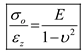# The homogeneous plate ABCD is subjected to a biaxial loading as shown that σz. = σ0 and that the change in length of the plate in the x direction must be zero, that is, ϵx = 0.Denoting by E the modulus of elasticity and by v Poisson's ratio, determine (a) the required magnitude of σx, (b) the ratio σ0/ϵz

Question-AnswerCategory: Strength of MaterialsThe homogeneous plate ABCD is subjected to a biaxial loading as shown that σz. = σ0 and that the change in length of the plate in the x direction must be zero, that is, ϵx = 0.Denoting by E the modulus of elasticity and by v Poisson's ratio, determine (a) the required magnitude of σx, (b) the ratio σ0/ϵz

The homogeneous plate ABCD is subjected to a biaxial loading as shown that σz. = σ0 and that the change in length of the plate in the x direction must be zero, that is, ϵx = 0.Denoting by E the modulus of elasticity and by v Poisson’s ratio, determine (a) the required magnitude of σx, (b) the ratio σ0/ϵz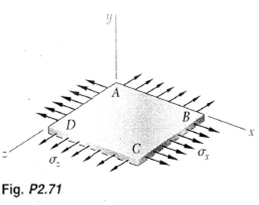Step No: 1

We are given that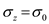Step No: 2

We know that strains in x and z directions are given by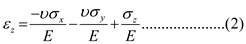Step No: 3

From (1) by putting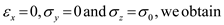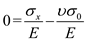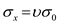Therefore required magnitude is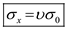Step No: 4

We putin equation (2),
So we have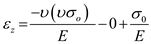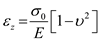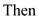Thus the required ratio is